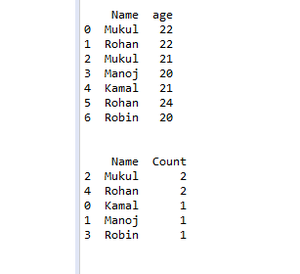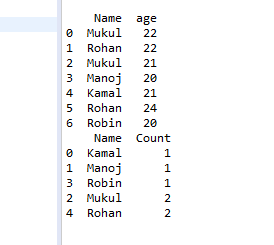# Sort Dataframe according to row frequency in Pandas

• Last Updated : 24 Feb, 2021

In this article, we will discuss how to use count() and sort_values() in pandas. So the count in pandas counts the frequency of elements in the dataframe column and then sort sorts the dataframe according to element frequency.

• count(): This method will show you the number of values for each column in your DataFrame.
• sort_values(): This method helps us to sort our dataframe. In this method, we pass the column and our data frame is sorted according to this column.

Example 1: Program to sort data frame in descending order according to the element frequency.

## Python

 `# import pandas``import` `pandas as pd`` ` `# create dataframe``df ``=` `pd.DataFrame({``'Name'``: [``'Mukul'``, ``'Rohan'``, ``'Mukul'``, ``'Manoj'``,``                            ``'Kamal'``, ``'Rohan'``, ``'Robin'``],``                    ` `                   ``'age'``: [``22``, ``22``, ``21``, ``20``, ``21``, ``24``, ``20``]})`` ` `# print dataframe``print``(df)`` ` `# use count() and sort()``df ``=` `df.groupby([``'Name'``])[``'age'``].count().reset_index(``  ``name``=``'Count'``).sort_values([``'Count'``], ascending``=``False``)`` ` `# print dataframe``print``(df)`

Output:Example 2: Program to sort data frame in ascending order according to the element frequency.

## Python

 `# import pandas``import` `pandas as pd`` ` `# create dataframe``df ``=` `pd.DataFrame({``'Name'``: [``'Mukul'``, ``'Rohan'``, ``'Mukul'``, ``'Manoj'``,``                            ``'Kamal'``, ``'Rohan'``, ``'Robin'``],``                    ` `                   ``'age'``: [``22``, ``22``, ``21``, ``20``, ``21``, ``24``, ``20``]})`` ` `# print dataframe``print``(df)`` ` `# use count() and sort()``df ``=` `df.groupby([``'Name'``])[``'age'``].count().reset_index(``  ``name``=``'Count'``).sort_values([``'Count'``], ascending``=``True``)`` ` `# print dataframe``print``(df)`

Output:My Personal Notes arrow_drop_up